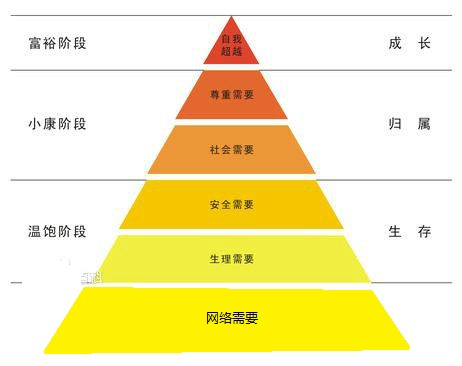## 体院校园网多设备联网解决方案（另一种思路）## CQRS 命令查询的责任分离

CQRS 全称：Command Query Responsibility Segregation

（另外，当然是存在有使用这个模式的框架，但他本身是个模式，不是框架）！

## 20171004 金秋动漫嘉年华 工作总结

emmmmmmm 每次写这个自己都是极度的无语，好在无语嘛~就是对自己有看法了，每次这样希望试一次进步

## 为什么计算机不善于处理小数

1 1×2^3 = 8
1 1×2^2 = 4
0 0x2^1 = 0
1 1×2^0 = 1
8 + 4 + 0 + 1 = 13

## C++字符串过滤

```
#include
#include
using namespace std;
string myFilter(string s);

int main(){
string s;
s = "wj哇nk.2啦啦啦q.qe.qe哈哈qw.dwd";
s = myFilter(s);
cout<='a'&&s[i]<='z')||
(s[i]>='A'&&s[i]<='Z')||
s[i]==' '||
s[i]=='\n'
){
for_return[j] = s[i];
j++;
}

}
return for_return;
}
```